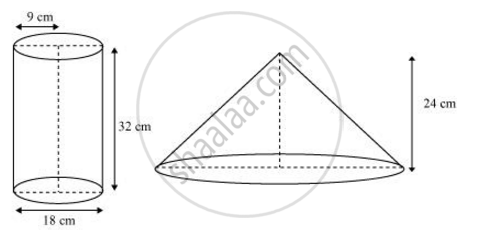Share
Notifications

View all notifications
Books Shortlist
Your shortlist is empty

# Solution for A cylindrical bucket, 32 cm high and with radius of base 18 cm, is filled with sand. This bucket is emptied on the ground and a conical heap of sand is formed. If the height of the conical heap is 24 cm. Find the radius and slant height of the heap. - CBSE Class 10 - Mathematics

Login
Create free account

Forgot password?
ConceptConversion of Solid from One Shape to Another

#### Question

A cylindrical bucket, 32 cm high and with radius of base 18 cm, is filled with sand. This bucket is emptied on the ground and a conical heap of sand is formed. If the height of the conical heap is 24 cm. Find the radius and slant height of the heap.

#### SolutionHeight (h1) of cylindrical bucket = 32 cm

Radius (r1) of circular end of bucket = 18 cm

Height (h2) of conical heap = 24 cm

Let the radius of the circular end of conical heap be r2.

The volume of sand in the cylindrical bucket will be equal to the volume of sand in the conical heap.

Volume of sand in the cylindrical bucket = Volume of sand in conical heap

pixxr_1^2xxh_1=1/3pixxr_2^2xxh_2

pixx18^2xx32=1/3pixxr_2^2xx24

pixx18^2xx32= 1.3pixxr_2^2xx24

r_2^2= (3xx18^2xx32)/24 = 18^2 xx 4

r2 = 18 x 2 = 36 cm

Slant height = sqrt(36^2+24^2) = sqrt(12^2xx(3^2+2^2)) = 12sqrt13

Therefore, the radius and slant height of the conical heap are 36 cm and

12sqrt13 respectively

Is there an error in this question or solution?

#### APPEARS IN

RD Sharma Solution for 10 Mathematics (2018 to Current)
Chapter 14: Surface Areas and Volumes
Q: 13
NCERT Solution for Mathematics Textbook for Class 10 (2014 to Current)
Chapter 13: Surface Areas and Volumes
Ex.13.3 | Q: 7 | Page no. 252

#### Video TutorialsVIEW ALL 

Solution A cylindrical bucket, 32 cm high and with radius of base 18 cm, is filled with sand. This bucket is emptied on the ground and a conical heap of sand is formed. If the height of the conical heap is 24 cm. Find the radius and slant height of the heap. Concept: Conversion of Solid from One Shape to Another.
S